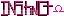-=+=- -=+=- -=+=- -=+=- -=+=- -=+=- -=+=- -=+=- -=+=- -=+=- -=+=- -=+=- -=+=- -=+=- -=+=- -=+=- -=+=- -=+=- -=+=- -=+=- -=+=- -=+=- -=+=- -=+=- -=+=- -=+=- -=+=- -=+=- -=+=- -=+=- (c) WidthPadding Industries 1987 0|339|0 -=+=- -=+=- -=+=- -=+=- -=+=- -=+=- -=+=- -=+=- -=+=- -=+=- -=+=- -=+=- -=+=- -=+=- -=+=- -=+=- -=+=- -=+=- -=+=- -=+=- -=+=- -=+=- -=+=- -=+=- -=+=- -=+=- -=+=- -=+=- -=+=- -=+=- Socoder -> On Topic -> Nice little surprise :) Posted : Tuesday, 15 January 2008, 14:19 NolanTake a look down the page -- especially you, Blanko. www.1up.com/do/feature?pager.offset=2&cId=3165201 -=-=- nolandc.comPosted : Tuesday, 15 January 2008, 14:46 Schererererwow, neat, congrats again blanko!Posted : Tuesday, 15 January 2008, 15:03 JayenkaiCool! Congrats Blanko! .. Always amazes me how very few of the games in those lists I've actually played! -=-=- ''Load, Next List!''Posted : Tuesday, 15 January 2008, 15:06 JL235Congratulations Blanko, your starting to make it! Posted : Tuesday, 15 January 2008, 15:48 power mouseycongratulations blanko, you don't have to make it.. continue to do what you like. for fun and learning, and then perhaps for profit. Posted : Tuesday, 15 January 2008, 19:04 magicmanthats freaking awesome! a huge congrats to you blanko! -=-=- Stuff... Yeah...Posted : Tuesday, 15 January 2008, 19:14 blanko1324Holy crap...Posted : Wednesday, 16 January 2008, 01:34 MetalManCongratulations blanko, great work manPosted : Wednesday, 16 January 2008, 05:34 HoboBenWell done mate!Posted : Wednesday, 16 January 2008, 10:12 moleAwesome!